When you count the outcomes of an experiment, you do not always observe all of the possible outcomes. For example, if you roll a six-sided die 10 times, it might be that the "1" face does not appear in those 10 rolls. Obviously, this situation occurs more frequently with small data than with large, although even for large data set, you might not observe rare events.

When conducting a statistical analysis, it is sometimes necessary to specify the unobserved categories. I have previously shown how to use the DATA step to add outcomes with zero counts and run PROC FREQ on the resulting data. I have also shown how to use the DATA step to merge observed data with expected values so that you can overlay a parametric density on a histogram.

In the SAS/IML language, you can use the LOC-ELEMENT technique to merge counts for observed outcomes into a vector of zeros. The result is the counts for all outcomes, where 0 indicates that an outcome is unobserved.

The following SAS/IML statements analyze 10 rolls of a six-sided die. The TABULATE subroutine counts the number of times each face appeared. The MergeCounts module inserts the observed counts into a vector.

```proc iml; /* Use LOC-ELEMENT technique to merge observed outcomes into all outcomes */ start MergeCounts(allVals, obsVals, counts); allCounts = j(nrow(allVals),ncol(allVals),0); /* array of 0s */ idx = loc(element(allVals, obsVals)); /* LOC-ELEMENT technique */ allCounts[idx] = counts; /* insert counts */ return( allCounts ); finish;   rolls = {4 6 4 6 5 2 4 3 3 6}; /* only 5 faces observed */ call tabulate(Vals, Counts, rolls);   allVals = 1:6; /* all outcomes */ allCounts = MergeCounts(allVals, Vals, Counts); print Counts[c=(char(Vals))] allCounts[c=(char(allVals))];```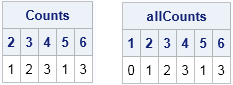Whereas the first table contains only the five observed faces, the second table lists all six faces of the die, with 0 indicating the "1" face was not observed.

In addition to the applications mentioned earlier, this technique is useful when you are computing goodness-of-fit statistics. For example, to compute the chi-square statistic for the null hypothesis that the die is fair (each face appears equally often), you need to form the difference between the observed and expected values for each face. The following statements implement a chi-square goodness-of-fit test:

```Expected = sum(allCounts) / 6; ChiSq = sum((allCounts-Expected)##2 / Expected); /* sum (O-E)^2/E over categories */ pval = 1 - cdf("chisquare", ChiSq, ncol(allVals)-1); /* p-value for test */ print ChiSq pval;```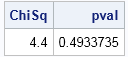Most statistical textbooks recommend that you not use the chi-square test if the expected counts are less than 5, as they are for this tiny data set. Nevertheless, this example illustrates the usefulness of adding unobserved outcome categories to data that contain the observed categories.

tags: Data Analysis

The post Merge observed outcomes into a list of all outcomes appeared first on The DO Loop."Daddy, help! Help me! Come quick!"

I heard my daughter's screams from the upstairs bathroom and bounded up the stairs two at a time. Was she hurt? Bleeding? Was the toilet overflowing?

When I arrived in the doorway, she pointed at the wall and at the floor. The wall was splattered with black nail polish. On the floor laid a broken bottle in an expanding pool of black ooze.

"It slipped," she sobbed.

As a parent, I know that there are times when I should not raise my voice. I knew intellectually that this was one of those times. But staring at that wall, seeing what I was seeing, I could not prevent myself from yelling.

"Oh my goodness!" I exclaimed. "Is that a logarithmic spiral?"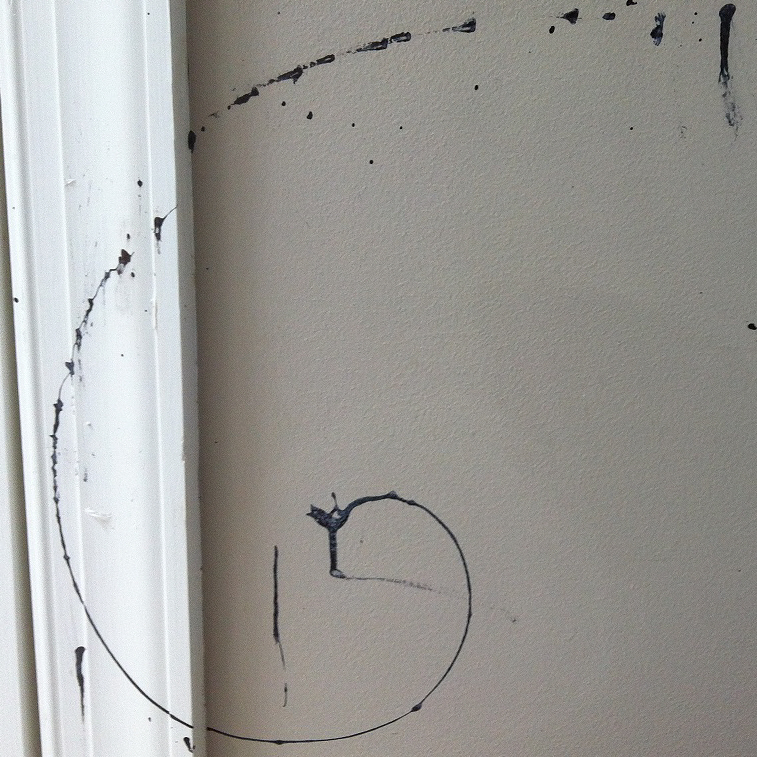There on the wall was a spiral formed from the drops of black nail polish as the bottle had spiraled out of my daughter's hands and ejected its contents. Logarithmic spirals appear in many natural phenomena, such as the spiral arms of galaxies, the bands of hurricanes, and the curve of a mollusk's shell. However, I had not expected to see such a beautiful spiral of splattered nail polish on my wall.

I took a picture of the spiral, and we began a futile attempt to clean the wall. The nail polish proved impossible to wipe from the wall, although we were able to clean the tile floor.

### How do you fit a logarithmic spiral?

As I was cleaning up the mess, my mind was racing. Is this really a logarithmic spiral? Could I construct a "spiral of best fit" that passes through the splattered drops?

I remembered that I once fit a circle to data by minimizing the radial distance between the data points and a circle with center (x0, y0) and radius R. This problem seems similar, but there are two differences. In the circle problem, I used the implicit equation of a circle (x-x0)2 + (y-y0)2 = R2. The logarithmic spiral is usually given explicitly as a polar (parametric) curve with the standard form R(θ) = a*exp(bθ), where a and b are unknown parameters. The second problem is that the spiral has multiple branches. For example, in the vertical direction there are several polar angles (π/2 and 5π/2), each with a different radius.

To construct a "spiral of best fit," you can do the following:

1. Choose a coordinate system and use the photo to find the (x,y) coordinates of points along the spiral curve.
2. Create an objective function whose minimum will produce parameters for the spiral that best fits the data. This problem has four parameters: The center of the spiral (x0, y0) and the two parameters a and b the amplitude and decay rate for the logarithmic spiral.
3. Fit the curve to the data and see if the splatter marks on the wall appear to be modeled by the curve.

### Finding points along the curve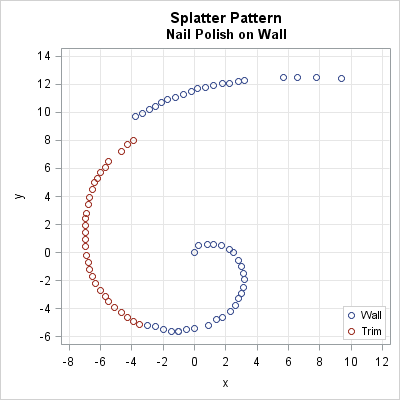As punishment for my daughter's carelessness, I told her that she had to help me input data from the photograph. She claimed that this punishment was "cruel and unusual," but she did it anyway.

I printed out the photo and used a ruler to draw a grid of lines 1 centimeter apart across the page. I chose the origin to be a big blob of nail polish at the end of spiral, about 1/3 up from the bottom of the image. I then marked points at 5 mm intervals along the curve and read out the coordinates (to the nearest 1 mm) as my daughter typed them into SAS. The resulting coordinates are shown in the scatter plot. A large portion of the nail polish landed on the door-frame molding, which is raised 1.5 cm above the wall. That explains the apparent discontinuity in the upper left portion of the graph.

### Create an objective function

To find the logarithmic spiral that best fit the data, you can minimize a function that computes the sum of the squared distances (in the radial direction) between the data and the predicted points along the spiral. Because the center of the spiral is unknown, you have fit four parameters: the center of the spiral (x0, y0) and the parameters a and b.

For given values (x0, y0), you can center the (x, y) coordinates by subtracting the coordinates of the center point. In a previous blog post, I showed how to obtain an increasing sequence of polar angles from the coordinates of (centered) data that are ordered along a parametric curve. Let θi be the polar angle that corresponds to the point (xi, yi). By using the fact that Euclidean and polar coordinates are related by x = R cos(θ) and y = R sin(θ), you can write down the predicted Euclidean coordinates of the logarithmic spiral:
ui = a cos(thetai) exp(b*thetai)
vi = a sin(thetai) exp(b*thetai)

The objective is therefore to minimize the radial distance between the predicted and observed values:
F(x0, y0, a, b) = Σi   (xi-ui)2 + (yi-vi)2

### Fit a logarithmic spiral to the data

To optimize the function I decided to use the trust region method (the NLPTR call) in SAS/IML software, just as I did when fitting a circle to data. Based on the scatter plot, I guessed that the center was approximately (x0, y0) = (1, -1), and that a ≈ 26 and b ≈ -0.5.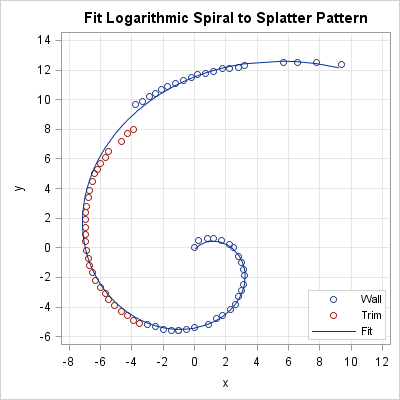You can download the complete SAS program, which uses SAS/IML to optimize the parameters. The logarithmic spiral that best fits the data is shown overlaid on the scatter plot of the data. The curve fits remarkably well, with the obvious exception of the nail polish that splattered on the door frame (trim) instead of on the wall. If that section of data were moved upwards by 1–1.5 cm, then the fit would be even better. For the record, the parameter estimates are (x0, y0) = (0.69, -1.06), a = 22.34, and b = -0.35.

### All models are wrong...including this one

As George Box famously said, "All models are wrong, but some are useful." However, this spiral model might be both wrong and useless.

I was so stunned by the appearance of this spiral on my wall that I didn't stop to think about whether a logarithmic spiral is a curve that can appear when a rotating bottle falls under the effects of gravity. The physics of falling objects is well understood.

Of course, I have no idea how the bottle was tumbling when it left my daughter's hands, nor do I know what angle the bottle was moving relative to the wall. Nevertheless, you can make some simplifying assumptions (bottle falling straight down, rotating in the horizontal plane as it falls,...) and compute the path that the nail polish would trace out in that simple situation. The physics-based model (not shown) also looks similar to the pattern on my wall. It's been a long time since freshman physics, and this is not a physics blog, so I invite the interested reader to download the SAS program and read my attempt to derive a physics-based model.

The main difference between the physics-based path and the logarithmic spiral is the function that is responsible for the radial decay of the spiral. In the logarithmic spiral, the decay function is the exponential function, which is a decreasing function that never reaches zero. In the physics-based model, the decay function is related to the distance between the bottle and the wall, which is a decreasing function that reaches zero in finite time. Any decreasing function will lead to a spiral, some of which have names. For example, a linear decreasing function is an Archimedean spiral.

I think that these data can be fit by many families of spirals because the bottle rotated only about 1.25 times before it hit the wall. To distinguish between the spirals, the data should show at least two full rotations so that the decay function can be more accurately modeled. However, no matter what model you choose, you can use the same optimization ideas to fit the parameters in the model.

To me it doesn't matter whether the spiral on my wall was logarithmic, Archimedean, or something else. It was cool to see, and I got to spend a little time talking about math with my daughter, which is a special treat. And, of course, I enjoyed the statistical modeling and SAS programming that I used to analyze these data.

Now I must stop writing. I have to paint that wall!

tags: Data Analysis, Math

The post The spiral of splatter appeared first on The DO Loop.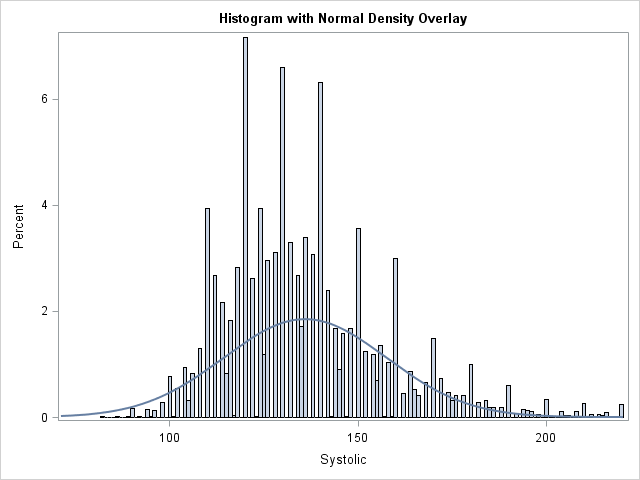I was reading a statistics book when I encountered a histogram that caught my eye. The histogram looked similar to the one at the left. It contained a normal density estimate overlaid on a histogram, but the height of the density curve seemed too short when compared to the heights of the bars.

The area under the density curve should equal the sum of the areas of the histogram bars. However, the height of the density curve in the histogram seems about half as high as the bars.

The graph in the book was created by using the SGPLOT procedure in SAS software. Could there be a bug in such a simple operation? I searched for the statements that created the graph and immediately noticed something suspicious. The SGPLOT statements included the NBINS=100 option in the HISTOGRAM statement, which sets the number of bins to 100. As in the graph at the top of this article, this resulted in many thin bars.

I have previously written about problems that can arise when you bin rounded data. It is well known that a histogram can display artifacts when the widths of the bins interact with data that are rounded. Common rounding factors include 1, 5, and powers of 10; commonly rounded variables include age, weight, and income.

I wondered if the histogram in the book was visualizing rounded data. The data were the pulse rates of individuals, measured in beats per minute. It is common for a nurse or technician to time a person's pulse for 30 seconds, and then multiply that value by 2 in order to convert to beats per minute. This can result in data that are multiples of 2. (Or multiples of 4, if the pulse is measured for 15 seconds.)

In the book, the range of the data was roughly from 40 beats per minute to 140 beats per minute. The NBINS=100 option therefore set the width of each bin to be approximately 1. If the values of the data are multiples of 2, every other bin could be empty!

A few experiments confirmed my conjecture. The sum of the areas of the histogram bars WAS the same as the area under the curve. However, every other bar was empty, which made the density curve look too short.

After I understood the problem, it was easy to reproduce it for other data sets. The following statements compute a histogram for the Systolic variable in the Sashelp.Heart data set, which measures blood pressure. Most values (93%) are multiples of two.

```title "Systolic Blood Pressure"; title2 "Default Bin Width"; proc sgplot data=sashelp.heart(where=(Systolic<=220)) noautolegend; histogram Systolic; density Systolic; run;   title2 "Bin Width Smaller Than the Rounding Unit"; proc sgplot data=sashelp.heart(where=(Systolic<=220)) noautolegend; histogram Systolic / binwidth=1; density Systolic; run;```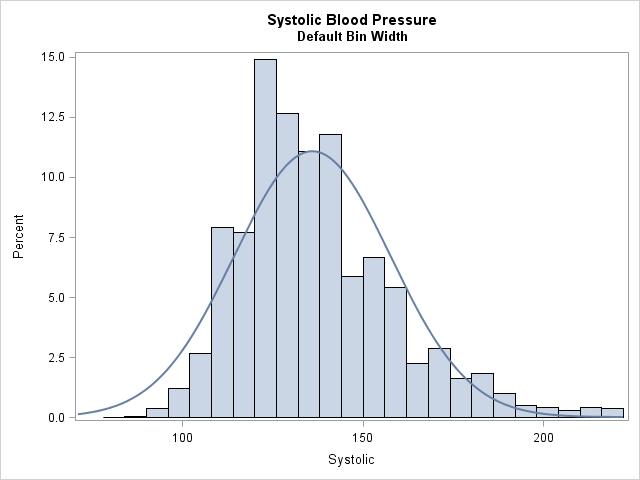The histogram with the normal density overlay looks fine for the default bin width. However, the second graph (shown at the top of the article) uses a bin width of 1 which results in empty bars in the histogram. Because the empty bars have zero area, the non-empty bars seem tall when compared with the normal curve.

It is easy to make this visual illusion disappear: Use a bin width that is at least as large as the rounding unit in the data. For example, changing the second PROC SGPLOT call to use BINWIDTH=2 makes the histogram look better.

So if your data are rounded, be careful when you choose a customized bin width. In particular, avoid using a bin width that is smaller than the rounding unit. A very small bin width can result in a histogram with many empty bars. When combined with a density estimate curve, the graph can be confusing to your readers.

The post The mystery of the density curve that was too short appeared first on The DO Loop.A SAS programmer asked for a list of SAS/IML functions that operate on the columns of an n x p matrix and return a 1 x p row vector of results. The functions that behave this way tend to compute univariate descriptive statistics such as the mean, median, standard deviation, and quantiles.

The following SAS/IML functions compute a statistic for each column of a matrix:

• COUNTMISS function: counts the number of missing values (use the "col" option)
• COUNTN function : counts the number of nonmissing values (use the "col" option)
• COUNTUNIQUE function: counts the number of unique values (use the "col" option)
• CV function: computes the sample coefficient of variation
• KURTOSIS function: computes the sample kurtosis
• MAD function: computes the univariate median absolute deviation
• MEAN function: computes sample means
• QNTL call: computes sample quantiles (returns multiple rows)
• QUARTILE function: computes the five-number summary (returns five rows: min, Q1, Q2, Q3, and max))
• SKEWNESS function: computes the sample skewness
• STD function: computes the sample standard deviation
• VAR function: computes the sample variance

You can also use subscript reduction operators to compute the following descriptive statistics for each column in a matrix X:

• X[+, ] returns the column sums (similar to the SUM function)
• X[#, ] returns the column products (similar to the PROD function)
• X[<>, ] returns the column maxima (similar to the MAX function)
• X[><, ] returns the column minima (similar to the MIN function)
• X[<:>, ] returns the index of the column maxima
• X[>:<, ] returns the index of the column minima
• X[##, ] returns the column sum of squares (similar to the SSQ function)

Lastly, elementwise operators (+, -, #, /, ##) in SAS/IML can operate on columns.

The post SAS/IML functions that operate on columns of a matrix appeared first on The DO Loop.Perhaps you saw the headlines earlier this week about the fact that it has been nine years since the last major hurricane (category 3, 4, or 5) hit the US coast. According to a post on the GeoSpace blog, which is published by the American Geophysical Union (AGU), researchers ran a computer model that "simulated the years 1950 through 2012 a thousand times to learn how often... virtual hurricanes managed to cross into 19 U.S. states ranging from Texas to Maine." Based on the results of their simulation study, the researchers concluded that it is highly unusual to have a nine-year period without major hurricanes: "Their analysis shows that the mean wait time before a nine-year hurricane drought occurred is 177 years." From that statement, I infer that the probability is 1/177 = 0.56% of having a nine-year "drought" in which major hurricanes do not hit the US.

Undoubtedly the simulation was very complex, since it incorporated sea temperatures, El Niño phenomena, and other climatic conditions. Is there an easy way to check whether their computation is reasonable? Yes! You can use historical data about major hurricanes to build a simple statistical model.

The National Hurricane Center keeps track of how many hurricanes of each Saffir-Simpson category hit the US mainland. The following SAS DATA step presents the data for each decade from 1851–2000:

```data MajorUS; input decade \$10. Count; datalines; 1851-1860 6 1861-1870 1 1871-1880 7 1881-1890 5 1891-1900 8 1901-1910 4 1911-1920 7 1921-1930 5 1931-1940 8 1941-1950 10 1951-1960 8 1961-1970 6 1971-1980 4 1981-1990 5 1991-2000 5 ; proc means data=MajorUS N sum mean var; var Count; run;```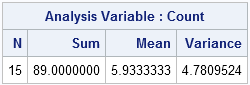The call to PROC MEANS shows that 89 storms hit the coastal US in those 15 decades. That produces an average rate of λ = 5.93 major storms per decade. The very simplest statistical model of events that occur over time is a homogeneous Poisson process, which assumes that a major storm hits the US at a constant rate of 5.93 times per decade. Obviously that model is at best an approximation, since in reality El Niño and other factors affect the rate.

Nevertheless, this simple statistical model produces a result that is consistent with the more sophisticated computer model. In the Poisson model, we expect 0.9*5.93 = 5.337 major storms to hit land every nine years. Then the probability of observing zero storms in a nine-year period is the Poisson density evaluated at 0:

```/* During a 9 year period, we expect 0.9*5.9333333 major hurricanes. */ /* What is probability of 0 major hurricanes in a nine-year period? */ data _null_; lambda = 0.9*5.9333333; p = pdf("Poisson", 0, lambda); /* P(X=0) */ put p; run;```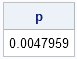According to the model, a nine-year "drought" of major hurricanes occurs less than 0.5% of the time. That means that it will occur about once every 200 years. Recall that the model used by the climate scientists estimated 0.56% and 177 years.

How rare is this "drought" of major hurricanes at landfall? It is more unusual than tossing a fair coin and having it land on heads seven times in a row: 0.57 = 0.78%.

Considering that you can do the Poisson calculations on a hand calculator in about two minutes, the estimates are amazingly close to the estimates from the more sophisticated simulation model. Although a simple statistical model is not a replacement for meteorological models, it is a simple way to show the rarity of the current nine-year drought.

The post Few major hurricanes have hit the US coast recently. Lucky us! appeared first on The DO Loop.Suppose that you compute the correlation matrix (call it R1) for a set of variables x1, x2, ..., x8. For some reason, you later want to compute the correlation matrix for the variables in a different order, maybe x2, x1, x7,..., x6. Do you need to go back to the data and compute the new correlation matrix (call it R2)? Or can you somehow obtain the new correlation matrix from the old one?

The answer, of course, is that you can create the new correlation matrix from the original matrix. After all, R1 contains all of the correlations between variables. The challenge is to figure out how to rearrange the numbers in R1 so that they form R2.

### Permutation matrices

Because the SAS/IML language has built-in support for matrix operations, it is a good tool for converting R1 into R2. Mathematically speaking, this is a classic situation in which a permutation matrix is useful. A permutation matrix is formed by permuting the rows of the identity matrix. I have written about using permutation matrices to create "Secret Santa" lists, where each person in a group gives a present to someone else in the group.

One of the nice properties of a permutation matrix is the ease with which you can permute rows and columns in a second matrix. If P is a permutation matrix and A is any square matrix, then the matrix product P*A permutes the rows of A. Similarly, the multiplication A*P permutes the columns of A.

The following SAS/IML module shows one way to generate a permutation matrix when the permutation is represented by a row vector:

```proc iml; /* create permutation matrix from a permutation, v, where v is a row vector */ start PermutationMatrix(v); return( I(ncol(v))[v, ] ); /* generate identity, then rorder rows */ finish;   perm = {2 1 7 3 5 8 4 6}; /* permutation of 1:8 */ P = PermutationMatrix(perm); print perm, P;```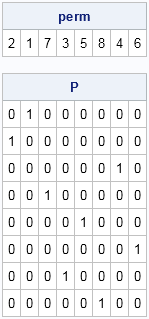The permutation matrix is a reordering of the rows of the identity matrix:

• The first row of P is the second row of the identity matrix because perm=2.
• The second row of P is the first row of the identity matrix because perm=1.
• The third row of P is the seventh row of the identity matrix because perm=7, and so forth.

### Reordering a correlation matrix

Let's see how the permutation matrix enables you to reorder a correlation matrix. The following statements compute a correlation matrix for eight variables in the Sashelp.Heart data set, which records measurements about patients in the Framingham Heart Study. The variables are listed in alphabetical order:

```/* list variables in alphabetical order */ varNames = {"AgeAtStart" "Cholesterol" "Diastolic" "Height" "MRW" "Smoking" "Systolic" "Weight"}; use Sashelp.Heart; read all var varNames into X; close;   R1 = corr(X); print R1[c=varNames r=varNames format=5.2];```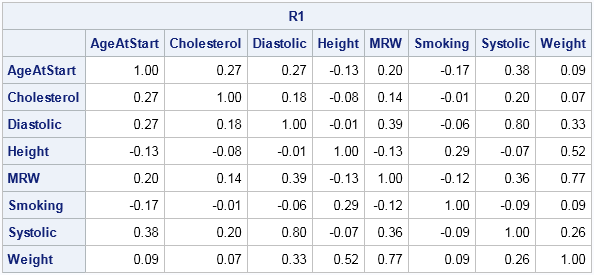The correlation matrix shows the correlations between the alphabetical list of variables. Applying a permutation such as perm will rearrange the variables into a different order. In the same way, you can apply the permutation matrix, P, to permute the rows and columns of R1. In turns out that the correct formula to use is R2 = P*R1*P', as shown below:

```Names = varNames[perm]; R2 = P*R1*P`; print R2[c=Names r=Names format=5.2];```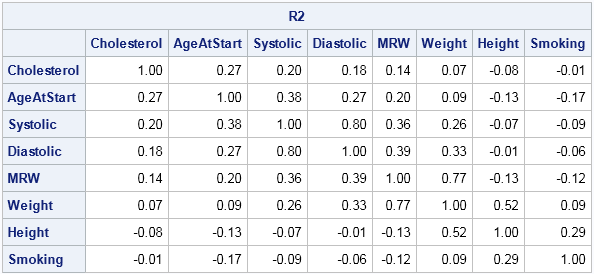You can verify that R2 is the correct correlation matrix for the reordered variables by repeating the correlation computation: corr(X[,perm]).

Why is this P*R1*P' the correct formula? Well, a permutation matrix is an example of an orthogonal matrix, so P` = P-1. That means that the formula specifies a similarity transformation between R1 and R2 that correspond to a change of bases. The new basis vectors are the specified permutation of the standard basis vectors.

Although I have demonstrated this technique for a correlation matrix, it applies to other matrices such as a covariance matrix or a distance matrix. It can be a useful technique when you rearrange the variable order so that similar variables are clustered together.

### An alternative solution

As demonstrated, you can use premultiplication by a permutation matrix to permute rows, and postmultiplication to permute columns. If you aren't comfortable with permutation matrices, there is an easy alternative way to create R2 by permuting the column and rows subscripts of R1:

```B = R1[,perm]; /* permute the columns */ R2 = B[perm,]; /* permute the rows */```

So whether you use the permutation matrix to permute the columns and rows of a matrix or whether you permute them directly by using subscripts, the SAS/IML language gives you an easy way to manipulate an array of new multivariate statistics to accommodate a change in the ordering of a list of variables.

The post Create and use a permutation matrix in SAS appeared first on The DO Loop.Imagine that you have one million rows of numerical data and you want to determine if a particular "target" value occurs. How might you find where the value occurs?

For univariate data, this is an easy problem. In the SAS DATA step you can use a WHERE clause or a subsetting IF statement to find the rows that contain the target value. In the SAS/IML language you can use the LOC function to find the rows.

For multivariate data, you can use a similar approach, except the code become messier. For example, suppose that you have a data set that contains five numeric variables and you want to test whether the 5-tuple (1, 2, 3, 4, 5) appears in the data. In the DATA step you might write the following statement:

`if x1=1 & x2=2 & x3=3 & x4=4 & x5=5 then ...;`

A general mathematical principle is that a "target problem" is equivalent to a root-finding problem. The standard mathematical trick is to subtract the target value and then find zeros of the resulting set of numbers. This trick provides the basis for writing a general SAS/IML programs that is vectorized and that can handle an arbitrary number of variables. The following statements generate a matrix that has one million rows and five columns. Each element is an integer 0 through 9.

```proc iml; call randseed(123); x = floor( 10*randfun({1e6 5}, "Uniform") ); /* matrix of values 0-9 */```

Suppose that you want to find whether there is a row that matches the target value {1 2 3 4 5}. The following statements subtract the target value from the data and then find all rows for which the difference is the zero vector:

```target = {1 2 3 4 5}; y = x - target; /* match target ==> y={0 0...0} */ count = (y=0)[,+]; /* count number of 0s in each row */ idx = loc(count=ncol(target)); /* rows that match target */ if ncol(idx)>0 then print "Target pattern found in " (ncol(idx)) " rows"; else print "Target pattern not found";```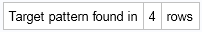The variable idx contains the row numbers for which the pattern occurs. For this random integer matrix, the target pattern appeared four times. Other random matrices might have six, 12, or 15 rows that match the target. It is easy to show that in a random matrix with one million rows, the target value is expected to appear 10 times.

The example in this article shows how to search a numerical matrix for rows that have a particular value. For character matrices, you can use the ROWCATC function in SAS/IML to concatenate the column values into a single vector of strings. You can then use the LOC function to find the rows that match the pattern.

tags: Data Analysis, Efficiency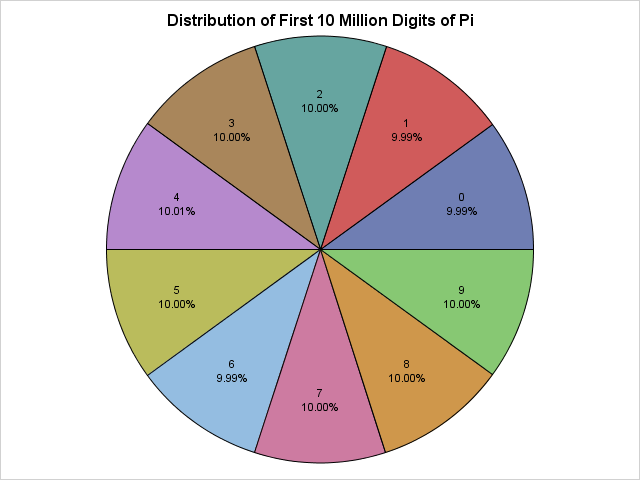Saturday, March 14, 2015, is Pi Day, and this year is a super-special Pi Day! This is your once-in-a-lifetime chance to celebrate the first 10 digits of pi (π) by doing something special on 3/14/15 at 9:26:53. Apologies to my European friends, but Pi Day requires that you represent dates with the month placed first in order to match the sequence 3.141592653....

Last year I celebrated Pi Day by using SAS to explore properties of the continued fraction expansion of pi. This year, I will examine statistical properties of the first 10 million digits of pi. In particular, I will show that the digits of pi exhibit statistical properties that are inherent in a random sequence of integers.

### Reading 10 million digits of pi

I have no desire to type in 10 million digits, so I will use SAS to read a text file at a Princeton University URL. The following statements use the FILENAME statement to point to the URL:

```/* read data over the internet from a URL */ filename rawurl url "http://www.cs.princeton.edu/introcs/data/pi-10million.txt" /* proxy='http://yourproxy.company.com:80' */ ;   data PiDigits; infile rawurl lrecl=10000000; input Digit 1. @@; Position = _n_; Diff = dif(digit); /* compute difference between adjacent digits */ run;   proc print data=PiDigits(obs=9); var Digit; run;```The PiDigits data set contains 10 million rows. The call to PROC PRINT displays the first few decimal digits, which are (skipping the 3) 141592653....

For other ways to use SAS to download data from the internet, search Chris Hemedinger's blog, The SAS Dummy for "PROC HTTP" and you will find several examples of how to download data from a URL.

### The distribution of digits of pi

You can run many statistical tests on these numbers. It is conjectured that the digits of pi are randomly uniformly distributed in the sense that the digits 0 through 9 appear equally often, as do pairs of digits, trios of digits, and so forth.

You can call PROC FREQ to compute the frequency distribution of the first 10 million digits of pi and to test whether the digits appear to be uniformly distributed:

```/* Are the digits 0-9 equally distributed? */ proc freq data = PiDigits; tables Digit/ chisq out=DigitFreq; run;```The frequency analysis of the first 10 million digits shows that each digit appears about one million times. A chi-square test indicates that the digits appear to be uniformly distributed. If you turn on ODS graphics, PROC FREQ also produces a deviation plot that shows that the deviations from uniformity are tiny.

### A "pi chart" of the distribution of the digits of pi

As an advocate of the #OneLessPie Chart Initiative, I am intellectually opposed to creating pie charts. However, I am going to grit my teeth and make an exception for this super-special Pi Day. You can use the Graph Template Language (GTL) to create a pie chart. Even simpler, Sanjay Matange has written a SAS macro that creates a pie chart with minimal effort. The following DATA step create a percentage variable and then calls Sanjay's macro:

```data DigitFreq; set DigitFreq; Pct = Percent/100; format Pct PERCENT8.2; run;   /* macro from http://blogs.sas.com/content/graphicallyspeaking/2012/08/26/how-about-some-pie/ */ %GTLPieChartMacro(data=DigitFreq, category=Digit, response=Pct, title=Distribution of First 10 Million Digits of Pi, DataSkin=NONE);```

The pie chart appears at the top of this article. It shows that the digits 0 through 9 are equally distributed.

### Any autocorrelation in the sequence?

In the DATA step that read the digits of pi, I calculated the difference between adjacent digits. You can use the SGPLOT procedure to create a histogram that shows the distribution of this quantity:

```proc sgplot data=PiDigits(obs=1000000); vbar Diff; run;```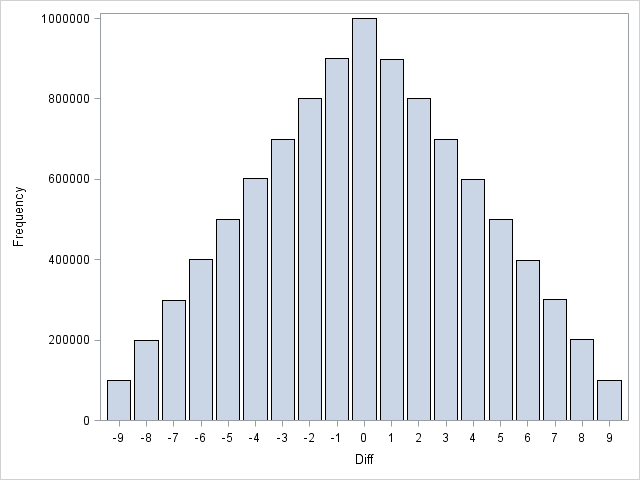That's a pretty cool triangular distribution! I won't bore you with mathematical details, but this shape arises when you examine the difference between two independent discrete uniform random variables, which suggests that the even digits of pi are independent of the odd digits of pi.

In fact, more is true. You can run a formal test to check for autocorrelation in the sequence of numbers. The Durbin-Watson statistic, which is available in PROC REG and PROC AUTOREG, has a value near 2 if a series of values has no autocorrelation. The following call to PROC AUTOREG requests the Durbin-Watson statistic for first-order through fifth-order autocorrelation for the first one million digits of pi. The results show that there is no detectable autocorrelation through fifth order. To the Durban-Watson test, the digits of pi are indistinguishable from a random sequence:

```proc autoreg data=PiDigits(obs=1000000); model Digit = / dw=5 dwprob; run;```### Are the digits of pi random?

Researchers have run dozens of statistical tests for randomness on the digits of pi. They all reach the same conclusion. Statistically speaking, the digits of pi seems to be the realization of a process that spits out digits uniformly at random.

Nevertheless, mathematicians have not yet been able to prove that the digits of pi are random. One of the leading researchers in the quest commented that if they are random then you can find in the sequence (appropriately converted into letters) the "entire works of Shakespeare" or any other message that you can imagine (Bailey and Borwein, 2013). For example, if I assign numeric values to the letters of "Pi Day" (P=16, I=9, D=4, A=1, Y=25), then the sequence "1694125" should appear somewhere in the decimal expansion of pi. I wrote a SAS program to search the decimal expansion of pi for the seven-digit "Pi Day" sequence. Here's what I found:

```proc print noobs data=PiDigits(firstobs=4686485 obs=4686491); var Position Digit; run;```There it is! The numeric representation of "Pi Day" appears near the 4.7 millionth decimal place of pi. Other "messages" might not appear in the first 10 million digits, but this one did. Finding Shakespearian sonnets and plays will probably require computing more digits of pi than the current world record.

The digits of pi pass every test for randomness, yet pi is a precise mathematical value that describes the relationship between the circumference of a circle and its diameter. This dichotomy between "very random" and "very structured" is fascinating! Happy Pi Day to everyone!Helping students to reason statistically is challenging enough without also having to provide in-class software instruction. “Practical Data Analysis with JMP, Second Edition” walks students through the process of analysis with JMP at their own speed at home, allowing faculty to devote class time to crucial or subtle statistical concepts […]I recently wrote about how to overlay multiple curves on a single graph by reshaping wide data (with many variables) into long data (with a grouping variable). The implementation used PROC TRANSPOSE, which is a procedure in Base SAS.

When you program in the SAS/IML language, you might encounter data in a matrix with many columns that you need to reshape. The SAS/IML language provides several functions for reshaping data:

In the PROC TRANSPOSE example, the data were reshaped by reading the data set in row-major order: The kth observation for the first variable is followed by the kth observation for the second, third, and fourth variables, and so forth. Consequently, the default behavior of the following WideToLong module is also to interleave the column values. However, the module also enables you to specify that the data should be stacked columnwise. That is, the reshaped data consists of all observations for the first variable, followed by all observations for the second variable, and so forth.

Some of the input variables might be numeric whereas others might be character, so the following module handles the X, Y, and grouping variables in separate matrices. The module returns three matrices: The long form of the Y matrix, the replicated X values, and the replicated grouping (or ID) variable. By convention, output variables are listed first in the argument list, as follows:

```proc iml; start WideToLong(OutY, OutX, OutG, /* output vars */ Y, X=T(1:nrow(Y)), Group=1:ncol(Y), stack=0); /* input vars */ p = ncol(Y); N = nrow(Y); cX = colvec(X); cG = colvec(Group); if stack then do; /* stack Y in column-major order */ OutY = shapecol(Y, 0, 1); /* stack cols of Y */ OutX = repeat(cX, p); /* replicate x */ OutG = colvec(repeat(cG, 1, N)); /* duplicate Group ID */ end; else do; /* DEFAULT: interleave Y in row-major order */ OutY = shape(Y, 0, 1); /* interleave cols of Y */ OutX = colvec(repeat(cX, 1, p)); /* replicate x */ OutG = repeat(cG, N); /* duplicate Group ID */ end; finish;```

Let's see how the module works by testing it on the same data as in the previous blog post. For the Sashelp.Tourism data, each observation is a year in the range 1966–1994. The year is stored in a variable named Year. (Technical note: The Year data is stored as a SAS date value, so the example uses the YEAR function to convert the date value to an integer.) The exchange rates for the British pound and the Spanish peseta are stored in separate variables named ExUK and ExSp, respectively. The following statements read the data into SAS/IML matrices, and then convert the data from wide to long form by calling the WideToLong routine:

```YNames = {ExUK ExSp}; GroupValues = {"UK" "Spain"}; XName = "Year";   use Sashelp.Tourism; read all var YNames into Y; read all var XName into X; close Sashelp.Tourism;   /* For these data, X is a SAS date value. Convert to integer. */ X = year(X); run WideToLong(ExchangeRate, Year, Country, /* output (long) */ Y, X, GroupValues); /* input (Y is wide) */```

As shown in the previous blog post, a useful application of reshaping data is that it is easy to overlay multiple line plots on a single graph. In the SAS/IML language, the SERIES statement creates series plots and supports the GROUP= option for overlaying multiple lines. The following statement creates a time series of the two exchange rates between 1966 and 1994:

```title "Exchange Rate Indices"; call series(Year, ExchangeRate) group=Country;```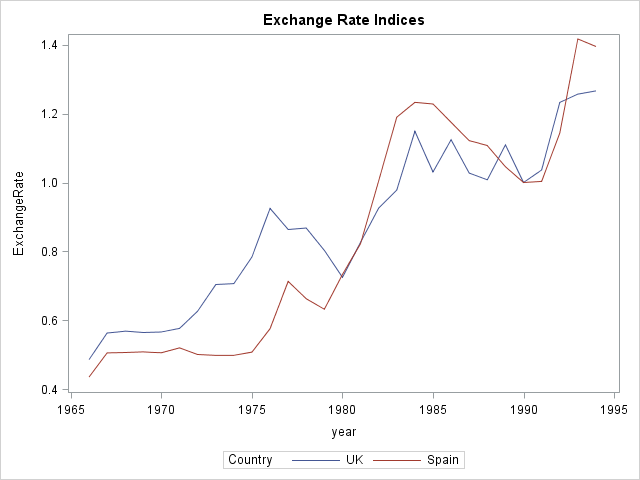By default, the data are reshaped in row-major order. To reshape in column-major order, specify the STACK=1 option, as follows:

```run WideToLong(ExchangeRate, Year, Country, /* output (long) */ Y, X, GroupValues) stack=1; /* input (Y is wide) */```